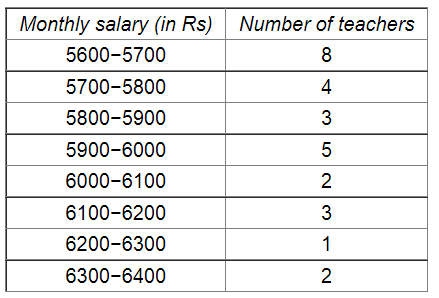# Draw a histogram to represent the following data:

Question:

Draw a histogram to represent the following data:Solution:

Taking class intervals as bases and the corresponding frequencies as heights, the rectangles can be constructed to obtain the histogram of the given data. The class intervals are represented along the x-axis and the frequencies along the y-axis on a suitable scale.

The histogram representing the given data is shown below: